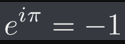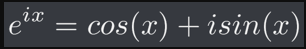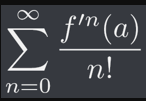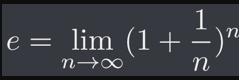# Deriving the world’s most Beautiful EquationEuler’s Identity is considered to be the most beautiful equation in mathematics by many.Euler’s Formula, the parent equation of Euler’s Identity

# Taylor Series

Before we dive deep into the derivation, we shall first understand what is a Taylor Series. This will be a bit complex. So if you do not want to read this, you can go ahead and skip to the part where I talk about Exponential series, it’s expansion and the expansion of sine and cosine functions.The Taylor expansion of a function.

# Exponential Series

An exponential series is a series in the form of e^nx where n can take any real values. The most famous exponential function is e^x. But what is this e?Definition of e as proposed by Bernoulli, and then quantified by EulerTaylor Expansion of exponential functions

# Series of Sine and Cosine

Sine and Cosine, otherwise known as sin and cos, are the two fundamental trigonometric functions.